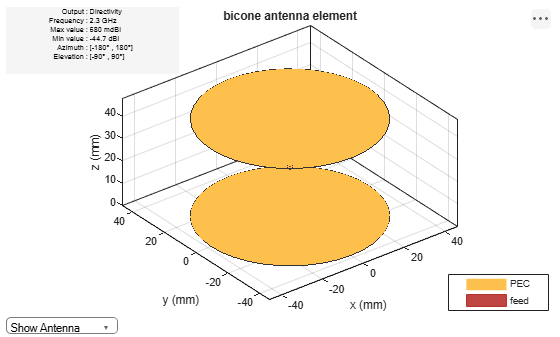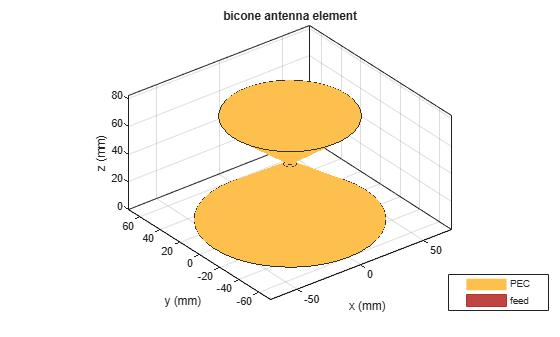# bicone

Create bicone antenna

## Description

The `bicone` object creates a bicone antenna. A bicone antenna consists of two symmetrical or asymmetrical cones separated by a small gap. The feed spans the gap and connects both the cones.

Bicone antennas are broadband omnidirectional antennas used for electronic support measure (ESM) applications. Bicone antennas are often used in electromagnetic interference (EMI) testing for immunity testing or emissions testing.## Creation

### Syntax

``ant = bicone``
``ant = bicone(Name,Value)``

### Description

example

````ant = bicone` creates a bicone antenna with dimensions for a resonant frequency of 2.3 GHz. The default bicone has a feedpoint at the apex of the top cone.```

example

````ant = bicone(Name,Value)` sets properties using one or more name-value pairs. For example, ```ant = bicone('Height',1)``` creates a bicone antenna with a cone of height 1 meter.```

## Properties

expand all

Vertical height of the cones, specified as a real-valued scalar in meters or a two-element vector with each element unit in meters. A scalar value creates two cones of the same height. The two-element vector can create two cones of different heights. In the two-element vector, the first element specifies the height of the top cone, and the second element specifies the height of the bottom cone.

Example: `'ConeHeight',[0.0215 0.0315]`

Example: `ant.ConeHeight = [0.0215 0.0315]`

Data Types: `double`

Radius at the apex of the cones, specified as a real-valued scalar in meters or a two-element vector with each element unit in meters. A scalar value creates two cones with the same narrow radius. A two-element vector can create two cones with different narrow radii. In the two-element vector, the first element specifies the narrow radius of the top cone, and the second element specifies the narrow radius of the bottom cone.

Example: ```'NarrowRadius',[6.3300e-04 0.0546]```

Example: ```ant.NarrowRadius = [6.3300e-04 0.0546]```

Data Types: `double`

Radius at the broad opening of the cones, specified as a real-valued scalar in meters or a two-element vector with each element unit in meters. A scalar value creates two cones with the same broad radius. A two-element vector can create two cones of different broad radii. In the two-element vector, the first element specifies the broad radius of the top cone, and the second element specifies the broad radius of the bottom cone.

Example: ```'BroadRadius',[8.3300e-04 0.0846]```

Example: ```ant.BroadRadius = [8.3300e-04 0.0846]```

Data Types: `double`

Gap between the two cones, specified as a real-valued scalar in meters.

Example: `'FeedHeight',0.0034`

Example: `ant.FeedHeight = 0.0034`

Data Types: `double`

Width of the feed, specified as a real-valued scalar in meters.

Example: `'FeedWidth',0.0050`

Example: `ant.FeedWidth = 0.0050`

Data Types: `double`

Type of the metal used as a conductor, specified as a metal material object. You can choose any metal from the `MetalCatalog` or specify a metal of your choice. For more information, see `metal`. For more information on metal conductor meshing, see Meshing.

Example: ```m = metal('Copper'); 'Conductor',m```

Example: ```m = metal('Copper'); ant.Conductor = m```

Lumped elements added to the antenna feed, specified as a lumped element object. You can add a load anywhere on the surface of the antenna. By default, the load is at the feed. For more information, see `lumpedElement`.

Example: `'Load',lumpedelement`. `lumpedelement` is the object for the load created using `lumpedElement`.

Example: ```ant.Load = lumpedElement('Impedance',75)```

Tilt angle of the antenna, specified as a scalar or vector with each element unit in degrees. For more information, see Rotate Antennas and Arrays.

Example: `'Tilt',90`

Example: `ant.Tilt = 90`

Example: `'Tilt',[90 90]`,`'TiltAxis',[0 1 0;0 1 1]` tilts the antenna at 90 degrees about the two axes defined by the vectors.

Note

The `wireStack` antenna object only accepts the dot method to change its properties.

Data Types: `double`

Tilt axis of the antenna, specified as:

• Three-element vector of Cartesian coordinates in meters. In this case, each coordinate in the vector starts at the origin and lies along the specified points on the X-, Y-, and Z-axes.

• Two points in space, each specified as three-element vectors of Cartesian coordinates. In this case, the antenna rotates around the line joining the two points in space.

• A string input describing simple rotations around one of the principal axes, 'X', 'Y', or 'Z'.

Example: `'TiltAxis',[0 1 0]`

Example: `'TiltAxis',[0 0 0;0 1 0]`

Example: `ant.TiltAxis = 'Z'`

Note

The `wireStack` antenna object only accepts the dot method to change its properties.

Data Types: `double`

## Object Functions

 `coneangle2size` Calculates equivalent cone height, broad radius, and narrow radius for cone `show` Display antenna or array structure; display shape as filled patch `axialRatio` Axial ratio of antenna `beamwidth` Beamwidth of antenna `charge` Charge distribution on metal or dielectric antenna or array surface `current` Current distribution on metal or dielectric antenna or array surface `design` Design prototype antenna or arrays for resonance around specified frequency `efficiency` Radiation efficiency of antenna `EHfields` Electric and magnetic fields of antennas; Embedded electric and magnetic fields of antenna element in arrays `impedance` Input impedance of antenna; scan impedance of array `mesh` Mesh properties of metal or dielectric antenna or array structure `meshconfig` Change mesh mode of antenna structure `optimize` Optimize antenna or array using SADEA optimizer `pattern` Radiation pattern and phase of antenna or array; Embedded pattern of antenna element in array `patternAzimuth` Azimuth pattern of antenna or array `patternElevation` Elevation pattern of antenna or array `rcs` Calculate and plot radar cross section (RCS) of platform, antenna, or array `returnLoss` Return loss of antenna; scan return loss of array `sparameters` Calculate S-parameter for antenna and antenna array objects `vswr` Voltage standing wave ratio of antenna

## Examples

collapse all

Create and view a default bicone antenna.

`ant = bicone`
```ant = bicone with properties: ConeHeight: 0.0215 NarrowRadius: 0.0013 BroadRadius: 0.0385 FeedHeight: 5.0000e-04 FeedWidth: 1.0000e-03 Conductor: [1x1 metal] Tilt: 0 TiltAxis: [1 0 0] Load: [1x1 lumpedElement] ```
`show(ant)`Plot the radiation pattern of the antenna at 2.3 GHz.

`pattern(ant,2.3e9)`Create a bicone antenna with asymmetrical cones.

```ant = bicone('NarrowRadius',[2e-3 4e-3],'BroadRadius',... [44.7e-3,60e-3],'ConeHeight',[33.7e-3 40e-3],'FeedHeight',... 1e-3,'FeedWidth',2e-3)```
```ant = bicone with properties: ConeHeight: [0.0337 0.0400] NarrowRadius: [0.0020 0.0040] BroadRadius: [0.0447 0.0600] FeedHeight: 1.0000e-03 FeedWidth: 0.0020 Conductor: [1x1 metal] Tilt: 0 TiltAxis: [1 0 0] Load: [1x1 lumpedElement] ```
`show(ant)`Calculate the impedance of the antenna over the frequency span of 500 MHz - 5 GHz.

`impedance(ant,linspace(0.5e9,5e9,51));`Kudpik, Rapin & Komask Meksamoot, Nipapon Siripon, and Sompol Kosulvit. "Design of a Compact Biconical Antenna for UWB Applications." 10.1109/ISPACS.2011.6146212.